# Differential gear ratio calculator

### Introduction

To be clear from the beginning, this article doesn’t explain how to calculate the gear ratio for a given set of gears. If you want to know how to calculate the gear ratio for a given pair of gears, read the article How to calculate a gear ratio ?

This article explains how the gear ratio for the differential is chosen / calculated / determined for a given vehicle, for which we know certain parameters.

When designing the powertrain of a new vehicle, regardless if it’s powered by an internal combustion engine or an electric machine, we need to decide what gear ratio we are going to have on the differential (also called final drive).

If you check different vehicles, you’ll see that they have different gear ratios for their differential. Some examples are described in the table below:

 Vehicle Maximum speed [kph] Wheel size Engine/Motor speed @ max power [rpm] Top gear [-] Final drive gear ratio [-] 19MY BMW M2 280 265/35 ZR 19 98Y 6250 0.85 3.46 21MY Ford Mustang Mach-E 180 225/60R18 12000 1.00 9.05

The data for the BMW was extracted from carfolio.com. The data for the Ford Mustang Mach-E was extracted from various sources on the internet. The maximum motor speed is an approximation, all the other data are provided by the manufacturer.

As you can see, there are different values for the gear ratio of the differential. The question which we’ll try to answer is: What gear ratio should my differential have? The answer for this question comes from physics, it’s not a guess.

To calculate the gear ratio of the differential we need to know the following:

• the maximum speed of the vehicle
• the engine speed for maximum power (if the vehicle is powered by an internal combustion engine) or the maximum speed of the motor (in the case of an electric vehicle)
• the gear (last gear of the gearbox) in which the maximum speed is obtained (this is usually the top gear for vehicles with high dynamic performance or the gear before top gear for the majority of vehicles)

The maximum speed of the vehicle is a preset value, it is a design target. Usually, when a manufacturer designs a new vehicle it will define what is its the top speed.

The rolling radius can be approximated by using the tyre symbol (e.g. 225/60R18). To understand how to calculate the rolling radius from the tyre symbol read the article How to calculate wheel radius.

If the vehicle is powered by an internal combustion engine then we need to know the characteristic of the engine in terms of engine speed at maximum power. This is needed because the maximum vehicle speed is obtained when the engine is spinning at maximum power (usually 5% above maximum power speed). Also we assume that we know in which gear of the gearbox the maximum speed is obtained.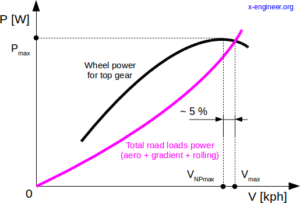Image: Maximum speed criteria function of power and road loads – internal combustion engine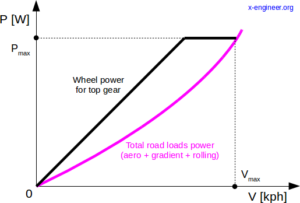Image: Maximum speed criteria function of power and road loads – electric motor

If the vehicle is powered by an electric motor, the maximum vehicle speed is obtained at maximum electric motor speed. Another difference to notice is that, usually, electric vehicle have only one gear reduction, which is the one on the differential (final drive). Since current electric vehicle do not have multi-step gearboxes, the top gear does not exist therefore in our calculation method we’ll consider its ratio to be 1.00 (which means that has no influence on speed and torque output).

The main assumption when calculating the differential gear ratio is that the maximum vehicle speed is obtained at maximum power engine speed (ICE) or maximum motor speed (electric motor). From this assumption we can draw a simplified kinematic schematic of the powertrain.

where:

NPmax [rpm] – is the engine speed at maximum power
ix [-] – gear ratio (gearbox) of the engaged gear
i0 [-] – differential gear ratio
NIN [rpm] – input speed of the differential
NOUT [rpm] – output speed of the differential
NVmax [rpm] – wheel speed at maximum vehicle speed

Observation: In the case of electric vehicles (EV) the pair of gears which forms the ix gear ratio doesn’t exist. The output of the electric motor is connected directly to the differential input shaft.

Go back

### Initial value of the gear ratio

Since there is a mechanical connection between the engine/motor and the wheel, with no slip, and assuming that the vehicle is travelling on a straight path, we can write speed balance of the differential:

$N_{IN} = i_{0} \cdot N_{OUT} \tag{1}$

The differential input speed can be written function of engine speed and the engaged gear of the gearbox:

$N_{IN} = \frac{N_{Pmax}}{i_{x}} \tag{2}$

Also, the differential output speed is equal with the wheel speed (assuming no tyre slip):

$N_{OUT} = N_{Vmax} \tag{3}$

The rotational speed of the wheel can be written function of the vehicle speed and wheel radius:

$N_{Vmax} = \omega_{max} \cdot \frac{30}{\pi} = v_{max} \cdot \frac{1}{r_{w}} \cdot \frac{30}{\pi} = \frac{V_{max}}{3.6} \cdot \frac{1}{r_{w}} \cdot \frac{30}{\pi} = 2.6526 \cdot \frac{V_{max}}{r_{w}} \tag{4}$

Replacing (4) in (3) and then (2) and (3) in (1) gives:

$\frac{N_{Pmax}}{i_{x}} = 2.6526 \cdot \frac{V_{max}}{r_{w}} \cdot i_{0} \tag{5}$

As discussed before, we’ll consider that in the case of a vehicle powered by an internal combustion engine, the maximum speed of the vehicle is obtained at a speed higher than the maximum power speed. Therefore we’ll introduce a maximum speed coefficient cNmax which will be multiplied with NPmax.

$\frac{c_{Nmax} \cdot N_{Pmax}}{i_{x}} = 2.6526 \cdot \frac{V_{max}}{r_{w}} \cdot i_{0} \tag{6}$

From (6) we can extract the equation which calculates the initial differential gear ratio:

$\bbox[#FFFF9D]{i_{0i} = \frac{c_{Nmax} \cdot N_{Pmax} \cdot r_{w}}{2.6526 \cdot i_{x} \cdot V_{max}}} \tag{7}$

Go back

### Final value of the gear ratio

Equation (7) gives the theoretical (initial, raw) value of the differential gear ratio. The actual (final) gear ratio is calculated from the number of teeth of the gear mesh. To calculate this we must first set the number of teeth of the input gear (pinion) zIN [-].

The minimum number of teeth for the input gear depends on the type of gears used for the differential.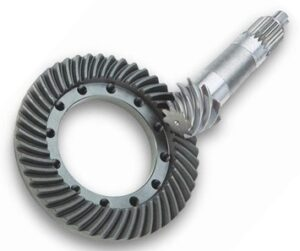Image: Hypoid bevel gearCredit: indiamart.com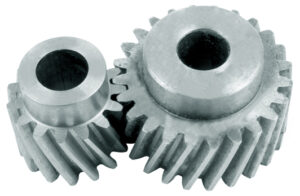Image: Parallel axis helical gearCredit: hpceurope.com

Depending on the layout of the powertrain, vehicles have different gear sets for the differential:

• longitudinal engine/motor vehicles have hypoid bevel gears, due to the fact that the axis of the gearbox’s output shaft is perpendicular on the axis of the drive axle
• transversal engine/motor vehicles have helical gears, due to the fact that axis of the gearbox’s output shaft is parallel with the axis of the drive axle

In general vehicles with internal combustion engines have both hypoid bevel and helical gears, depending on the location and engine mount. Electric vehicles however have parallel axis helical gears for the differential since the axis of the motor is parallel with the axis of the drive axle.

For the hypoid bevel gear, the minimum number of teeth depends on the value of the gear ratio . The input gear is called pinion gear and has less teeth than the output gear.

 i0 [-] 2.5 3 4 5 6-7 >7 zIN [-] *15 *12 9 7 5 5

* for gear ratios below 3 the pinion gear can have 11 teeth or more

For helical gear, the minim number of teeth of the input gear can be any number between 14 – 17 . In the case of electric vehicles, if the final drive gear ratio is high (> 10.00) the simple gear mechanism can be split into two-stage gear train. This is usually done to limit the size of the output gear.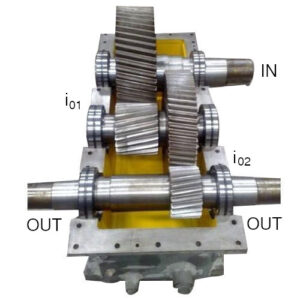Image: Two stage helical gearset – 3 parallel axesCredit: indiamart.com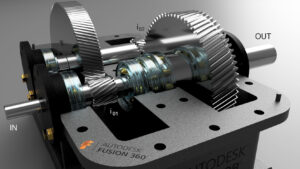Image: Two stage helical gearset – 2 concentric axesCredit: gallery.autodesk.com

In the case of the two-stage gear set, the overall gear ratio will be the product between the intermediate gear ratios:

$i_{0} = i_{01} \cdot i_{02} \tag{8}$

For simplicity, in our calculation examples we are only going to consider simple gear sets for the differential.

The number of teeth of the differential gears are just a rough estimations (starting values). The final number will depend on several factors like: geometry, size, reliability, manufacturing process, etc.

After deciding the type of gear set, we can choose the number of teeth for the input (pinion) gear. As example, we can choose several value, for example:

$z_{IN} = 11, 12, 13$

The next step is to calculate the number of teeth of the output gear zOUT [-], which is the product between the number of teeth of the input gear and initial gear ratio of the differential.

$z_{OUT} = z_{IN} \cdot i_{0i} \tag{9}$

Equation (9) will give a real number for the number of teeth of the output gear. We’ll need to round this number to the nearest integer number. For example if zOUT = 73.234 it will be rounded to 73, if zOUT = 81.74 it will be rounded to 82.

Go back

### Gear ratio correction

Since zOUT will be different from the initial calculated value, we need to recalculate the gear ratio with the rounded value of zOUT.

$i_{0f} = \frac{z_{OUT}}{z_{IN}} \tag{10}$

With the recalculated gear ratio we can also recalculate the maximum speed of the vehicle, to see the deviations from the initial value. If we reorder equation (7), we’ll get the expression of the maximum speed as:

$V_{maxf} = \frac{c_{Nmax} \cdot N_{Pmax} \cdot r_{w}}{2.6526 \cdot i_{x} \cdot i_{0}} \tag{11}$

To make sure that the maximum speed requirement is met, we’ll bring forward only the gear ratios for which the maximum speed is reached or exceeded.

The final criteria of the gear ratio calculation is the relative error between the initial value of the gear ratio and the final values after the rounding of zOUT.

$e_{i_{0}} = \left | 100 \cdot \frac{i_{0i} – i_{0f}}{i_{0i}} \right | \text{ [%]} \tag{12}$

The gear ratio which has the smallest relative error is going to be chosen as final value for the differential (final drive).

Go back

### Logical diagram

All the steps for the calculation of the differential gear ratio are summarised in the logical diagram below.

Go back

### Example for an internal combustion vehicle

For the BMW vehicle from the table above let’s calculate the final drive ratio (differential) based on the input data. Since we have the real gear ratio value already provided, we can compare it with our calculated value to validate the calculation process.

Step 1. Calculate the wheel radius, see the article How to calculate wheel radius for details.

$r_{w} = 0.334$

Step 2. Calculate the initial gear ratio using equation (7).

$i_{0i} = \frac{1.05 \cdot 6250 \cdot 0.334}{2.6526 \cdot 0.85 \cdot 280} = 3.472$

As you can see the initial calculated value of 3.472 is very close to the manufacturer value of 3.46.

Step 3. Set 4 values for the number of teeth for the input gear (pinion).

$z_{IN} = \text{11, 12, 13, 14}$

Step 4. Calculate the number of teeth for the output gear using equation (9) and round towards nearest integer.

$z_{OUT} = \text{38, 42, 45, 49}$

Step 5. Recalculate the differential gear ratio using equation (10).

$i_{0f} = \text{3.455, 3.5, 3.462, 3.5}$

Step 6. Recalculate the maximum vehicle speed using equation (11) and round towards nearest integer.

$V_{maxf} = \text{281, 278, 281, 278}$

As you can see, for the gear ratio of 3.5 the maximum vehicle speed is below initial target of 280 kph. For this reason we are not going to consider the gear ratio of 3.5 for our final decision.

Step 7. Calculate the relative error of the final gear ratios 3.455 and 3.462 compared with the initial value of 3.472 using equation (12).

$e_{i_{0}} = \text{0.4998, 0.2984}$

The smallest error is for the gear ratio of 3.462 therefore it’s going to be chosen for the vehicle as a design parameter.

Step 8. List the final parameters for the gear ratio calculation.

$\begin{split} z_{IN} &= 13\\ z_{OUT} &= 45\\ i_{0} &= 3.462 \end{split}$

As you can see our calculated value (up to the second decimal) is exactly the same as the one provided by the manufacturer which proves that the calculation method is correct.

Observation: This calculation methodology is mainly taking into account the maximum speed criteria and some gear geometry criteria. In reality, the decision regarding the number of teeth for the gear set might take into account other factors like: manufacturing, wear, reliability, geometry, etc. and the final result of the gear ratio might differ.

Go back

### Example for an electric vehicle

For the Ford Mach-E vehicle from the table above let’s calculate the final drive ratio (differential) based on the input data. Since we have the real gear ratio value already provided, we can compare it with our calculated value to validate the calculation process.

Step 1. Calculate the wheel radius, see the article How to calculate wheel radius for details.

$r_{w} = 0.364$

Step 2. Calculate the initial gear ratio using equation (7).

$i_{0i} = \frac{1.00 \cdot 12000 \cdot 0.364}{2.6526 \cdot 1.00 \cdot 180} = 9.148$

As you can see the initial calculated value of 9.148 is relatively close to the manufacturer value of 9.050.

Step 3. Set 4 values for the number of teeth for the input gear (pinion).

$z_{IN} = \text{14, 15, 16, 17}$

Step 4. Calculate the number of teeth for the output gear using equation (9) and round towards nearest integer.

$z_{OUT} = \text{128, 137, 146, 156}$

Step 5. Recalculate the differential gear ratio using equation (10).

$i_{0f} = \text{9.143, 9.133, 9.125, 9.176}$

Step 6. Recalculate the maximum vehicle speed using equation (11) and round towards nearest integer.

$V_{maxf} = \text{180, 180, 180, 179}$

As you can see, for the gear ratio of 9.176 the maximum vehicle speed is below initial target of 180 kph. For this reason we are not going to consider the gear ratio of 9.176 for our final decision.

Step 7. Calculate the relative error of the final gear ratios of 9.143, 9.133, 9.125 and compared with the initial value of 9.148 using equation (12).

$e_{i_{0}} = \text{0.059, 0.163, 0.254}$

The smallest error is for the gear ratio of 9.143 therefore it’s going to be chosen for the vehicle as a design parameter.

Step 8. List the final parameters for the gear ratio calculation.

$\begin{split} z_{IN} &= 14\\ z_{OUT} &= 128\\ i_{0} &= 9.143 \end{split}$

As you can see our calculated value of the gear ratio is relatively closer to the one published by the manufacturer.

Go back

### Calculator

You can try different parameters of the vehicle and get the differential gear ratio using the calculator below.

 Vmax [kph] rw [m] ix [-] NPmax [rpm] cNmax [rpm] i0i [-] = 2.802 zIN1 [-] = zOUT1 [-] = 25 i01f [-] = 2.778 ei01 [%] = 0.864 Vmaxf1 [kph] = 252.2 zIN2 [-] = zOUT2 [-] = 31 i02f [-] = 2.818 ei02 [%] = 0.578 Vmaxf2 [kph] = 248.6 zIN1 [-] = zOUT3 [-] = 36 i03f [-] = 2.769 ei03 [%] = 1.169 Vmaxf3 [kph] = 253.0

A Scilab script which automatically calculates the differential gear ratio is available on the Patreon page.

Go back

 Aurel P. Stoicescu, Proiectarea performantelor de tractiune si de consum ale automobilelor, Editura Tehnica, Bucuresti, 2007.